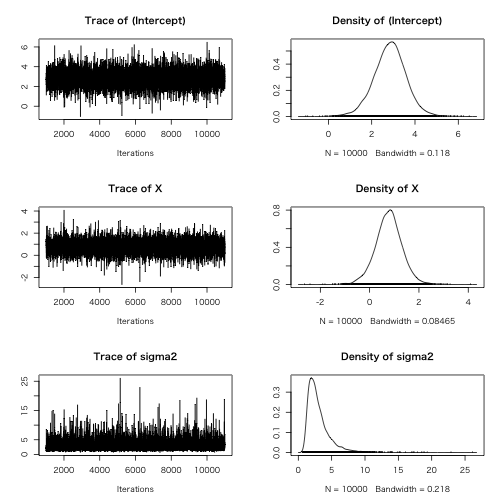# MCMCpack: Markov chain Monte Carlo (MCMC) Package``````> library(MCMCpack)
``````

バージョン: 1.3.3

`BayesFactor` Create an object of class BayesFactor from MCMCpack output
`Dirichlet` The Dirichlet Distribution
`HMMpanelFE` Markov Chain Monte Carlo for the Hidden Markov Fixed-effects Model
`HMMpanelRE` Markov Chain Monte Carlo for the Hidden Markov Random-effects Model
`InvGamma` The Inverse Gamma Distribution
`InvWishart` The Inverse Wishart Distribution
`MCMCSVDreg` Markov Chain Monte Carlo for SVD Regression
`MCMCbinaryChange` Markov Chain Monte Carlo for a Binary Multiple Changepoint Model
`MCMCdynamicEI` Markov Chain Monte Carlo for Quinn's Dynamic Ecological Inference Model
`MCMCdynamicIRT1d` Markov Chain Monte Carlo for Dynamic One Dimensional Item Response Theory Model
`MCMCfactanal` Markov Chain Monte Carlo for Normal Theory Factor Analysis Model
`MCMChierEI` Markov Chain Monte Carlo for Wakefield's Hierarchial Ecological Inference Model
`MCMChlogit` Markov Chain Monte Carlo for the Hierarchical Binomial Linear Regression Model using the logit link function
`MCMChpoisson` Markov Chain Monte Carlo for the Hierarchical Poisson Linear Regression Model using the log link function
`MCMChregress` Markov Chain Monte Carlo for the Hierarchical Gaussian Linear Regression Model
`MCMCintervention` Markov Chain Monte Carlo for a linear Gaussian Multiple Changepoint Model
`MCMCirt1d` Markov Chain Monte Carlo for One Dimensional Dimensional Item Response Theory Model, Covariates Predicting Latent Ideal Point (Ability)
`MCMCirtHier1d` Markov Chain Monte Carlo for Hierarchical One Dimensional Item Response Theory Model, Covariates Predicting Latent Ideal Point (Ability)
`MCMCirtKd` Markov Chain Monte Carlo for K-Dimensional Item Response Theory Model
`MCMCirtKdHet` Markov Chain Monte Carlo for Heteroskedastic K-Dimensional Item Response Theory Model
`MCMCirtKdRob` Markov Chain Monte Carlo for Robust K-Dimensional Item Response Theory Model
`MCMClogit` Markov Chain Monte Carlo for Logistic Regression
`MCMCmetrop1R` Metropolis Sampling from User-Written R function
`MCMCmixfactanal` Markov Chain Monte Carlo for Mixed Data Factor Analysis Model
`MCMCmnl` Markov Chain Monte Carlo for Multinomial Logistic Regression
`MCMCoprobit` Markov Chain Monte Carlo for Ordered Probit Regression
`MCMCoprobitChange` Markov Chain Monte Carlo for Ordered Probit Changepoint Regression Model
`MCMCordfactanal` Markov Chain Monte Carlo for Ordinal Data Factor Analysis Model
`MCMCpoisson` Markov Chain Monte Carlo for Poisson Regression
`MCMCpoissonChange` Markov Chain Monte Carlo for a Poisson Regression Changepoint Model
`MCMCprobit` Markov Chain Monte Carlo for Probit Regression
`MCMCprobitChange` Markov Chain Monte Carlo for a linear Gaussian Multiple Changepoint Model
`MCMCquantreg` Bayesian quantile regression using Gibbs sampling
`MCMCregress` Markov Chain Monte Carlo for Gaussian Linear Regression
`MCMCregressChange` Markov Chain Monte Carlo for a linear Gaussian Multiple Changepoint Model
`MCMCresidualBreakAnalysis` Break Analysis of Univariate Time Series using Markov Chain Monte Carlo
`MCMCtobit` Markov Chain Monte Carlo for Gaussian Linear Regression with a Censored Dependent Variable
`MCbinomialbeta` Monte Carlo Simulation from a Binomial Likelihood with a Beta Prior
`MCmultinomdirichlet` Monte Carlo Simulation from a Multinomial (with known variance) with a Normal Prior
`MCnormalnormal` Monte Carlo Simulation from a Normal Likelihood (with known variance) with a Normal Prior
`MCpoissongamma` Monte Carlo Simulation from a Poisson Likelihood with a Gamma Prior Nethvote
`mptable` Calculate the marginal posterior probabilities of predictors being included in a quantile regression model.
`Nethvote` Dutch Voting Behavior in 1989
`NoncenHypergeom` The Noncentral Hypergeometric Distribution
`PErisk` Political Economic Risk Data from 62 Countries in 1987
`PostProbMod` Calculate Posterior Probability of Model
`Rehnquist` U.S. Supreme Court Vote Matrix, Rehnquist Court (1994-2004)
`SSVSquantreg` Stochastic search variable selection for quantile regression
`Senate` 106th U.S. Senate Roll Call Vote Matrix
`SupremeCourt` U.S. Supreme Court Vote Matrix
`Wishart` The Wishart Distribution
`choicevar` Handle Choice-Specific Covariates in Multinomial Choice Models
`dtomogplot` Dynamic Tomography Plot
`make.breaklist` Vector of break numbers
`mptable` Calculate the marginal posterior probabilities of predictors being included in a quantile regression model.
`plot.qrssvs` Plot output from quantile regression stochastic search variable selection (QR-SSVS).
`plotChangepoint` Posterior Density of Regime Change Plot
`plotIntervention` Plot of intervention analysis
`plotState` Changepoint State Plot
`procrustes` Procrustes Transformation
`read.Scythe` Read a Matrix from a File written by Scythe
`summary.qrssvs` Summarising the results of quantile regression stochastic search variable selection (QR-SSVS).
`testpanelGroupBreak` A Test for the Group-level Break using a Multivariate Linear Regression Model with Breaks
`testpanelSubjectBreak` A Test for the Subject-level Break using a Unitivariate Linear Regression Model with Breaks
`tomogplot` Tomography Plot
`topmodels` Shows an ordered list of the most frequently visited models sampled during quantile regression stochastic search variable selection (QR-SSVS).`
`vech` Extract Lower Triangular Elements from a Symmetric Matrix
`write.Scythe` Write a Matrix to a File to be Read by Scythe
`xpnd` Expand a Vector into a Symmetric Matrix

## MCMCregress

### Arguments

• formula
• data
• burnin
• mcmc
• thin
• verbose
• seed
• beta.start
• b0
• B0
• c0
• d0
• sigma.mu
• sigma.var
• marginal.likelihood
• ...
``````> line   <- list(X = c(-2, -1, 0, 1, 2),
+                Y = c(1, 3, 3, 3, 5))
> line %>% lm(Y ~ X, data = .) %>% broom::tidy()
> line %>% MCMCregress(formula   = Y ~ X,
+                           b0        = 0,
+                           B0        = 0.1,
+                           sigma.mu  = 5,
+                           sigma.var = 25,
+                           data      = .,
+                           verbose   = 1000) -> posterior
> summary(posterior)
> plot(posterior)
``````